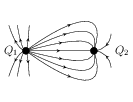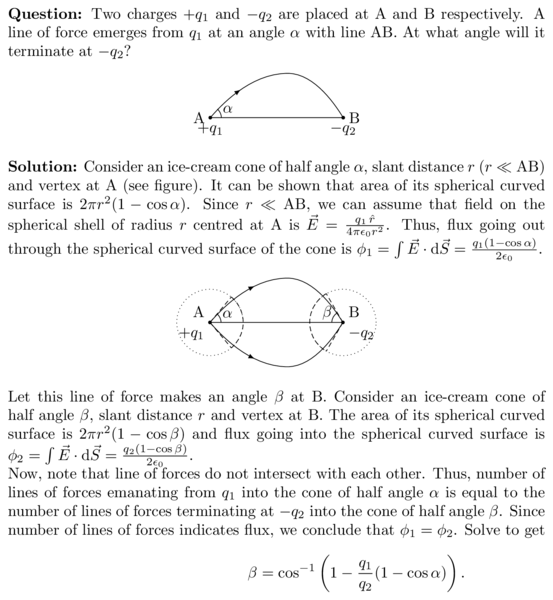# Electric Field Lines

## Problems from IIT JEE

Problem (IIT JEE 2010):A few electric field lines for a system of two charges $Q_1$ and $Q_2$ fixed at two different points on the $x$-axis are shown in the figure. These lines suggest that,

1. $|Q_1|>|Q_2|$.
2. $|Q_1|<|Q_2|$.
3. at a finite distance to the left of $Q_1$ the electric field is zero.
4. at a finite distance to the right of $Q_2$ the electric field is zero.

Solution: From the direction of electric field lines, $Q_1$ is positive and $Q_2$ is negative. The density of electric field lines (which is an indication of flux) is more around $Q_1$ in comparison to that around $Q_2$. In other words, flux $\phi_1$ through a small sphere containing $Q_1$ is more than flux $\phi_2$ through a similar sphere containing $Q_2$. From Gauss's law, flux $\phi=q_\text{enc}/\epsilon_0$. Thus, $\phi_1>\phi_2$ implies $|Q_1|>|Q_2|$. The electric field at a distance $x$ towards right of $Q_2$ is given by \begin{alignat}{2} &|\vec{E}|=\frac{1}{4\pi\epsilon_0}\frac{Q_1}{(d+x)^2}-\frac{1}{4\pi\epsilon_0}\frac{Q_2}{x^2},\nonumber \end{alignat} where $d$ is separation between $Q_1$ and $Q_2$. Since $Q_1>Q_2$, the $|\vec{E}|$ becomes zero for some finite $x$. The readers are encouraged to show that there are two such points given by \begin{align} &x_1=\frac{Q_2+\sqrt{Q_1Q_2}}{Q_1-Q_2}d, &&x_2=\frac{Q_2-\sqrt{Q_1Q_2}}{Q_1-Q_2}d.\nonumber \end{align} Also, show that the electric field is non-zero at all finite distances towards left of $Q_1$. Draw the electric field lines in entire region. Thus, correct options are A and D.

Problem: Two charges $+q_1$ and $-q_2$ are placed at A and B respectively. A line of force emerges from $q_1$ at an angle $\alpha$ with line AB. At what angle will it terminate at $-q_2$?Solution: Consider an ice-cream cone of half angle $\alpha$, slant distance $r$ ($r\ll\mathrm{AB}$) and vertex at A (see figure). It can be shown that area of its spherical curved surface is $2\pi r^2(1-\cos\alpha)$. Since $r\ll \mathrm{AB}$, we can assume that field on the spherical shell of radius $r$ centred at A is $\vec{E}=\frac{q_1\,\hat{r}}{4\pi\epsilon_0 r^2}$. Thus, flux going out through the spherical curved surface of the cone is $\phi_1=\int \vec{E}\cdot\mathrm{d}\vec{S}=\frac{q_1(1-\cos\alpha)}{2\epsilon_0}$.Let this line of force makes an angle $\beta$ at B. Consider an ice-cream cone of half angle $\beta$, slant distance $r$ and vertex at B. The area of its spherical curved surface is $2\pi r^2(1-\cos\beta)$ and flux going into the spherical curved surface is $\phi_2=\int \vec{E}\cdot\mathrm{d}\vec{S}=\frac{q_2(1-\cos\beta)}{2\epsilon_0}$.

Now, note that line of forces do not intersect with each other. Thus, number of lines of forces emanating from $q_1$ into the cone of half angle $\alpha$ is equal to the number of lines of forces terminating at $-q_2$ into the cone of half angle $\beta$. Since number of lines of forces indicates flux, we conclude that $\phi_1=\phi_2$. Solve to get \begin{align} \beta=\cos^{-1}\left(1-\frac{q_1}{q_2}(1-\cos\alpha)\right).\nonumber \end{align}